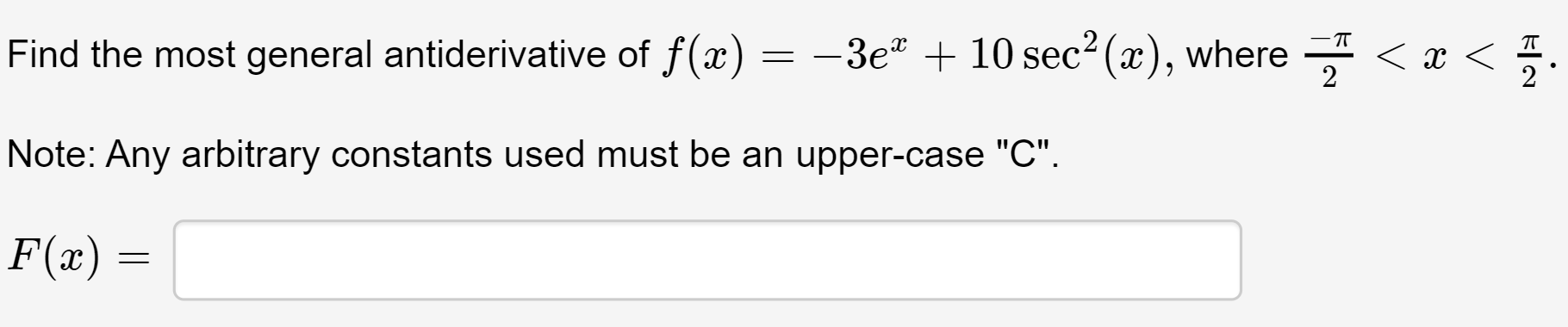# Find the most general antiderivative of f(x)= -3e" + 10 sec2 (x), where - < x <2Note: Any arbitrary constants used must be an upper-case "C".F(x) =

Question
8 viewshelp_outlineImage TranscriptioncloseFind the most general antiderivative of f(x) = -3e" + 10 sec2 (x), where - < x < 2 Note: Any arbitrary constants used must be an upper-case "C". F(x) = fullscreen
check_circle

Step 1

According to the given information it is required to calculate the antiderivative of the function:

Step 2

The antiderivative of the functi...

### Want to see the full answer?

See Solution

#### Want to see this answer and more?

Solutions are written by subject experts who are available 24/7. Questions are typically answered within 1 hour.*

See Solution
*Response times may vary by subject and question.
Tagged in
MathCalculus

### Integration